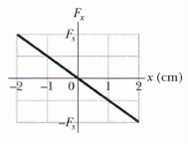### Create an Account

Home / Questions / Figure gives spring force Fx versus position x for the spring-block arrangement of Fig 7 1...

# Figure gives spring force Fx versus position x for the spring-block arrangement of Fig 7 11 The scale is set by F 160 0 N. We release the block at x = 12 cm How much work does the spring do on

Figure gives spring force Fx versus position x for the spring-block arrangement of Fig. 7 -11 The scale is set by F = 160.0 N. We release the block at x = 12 cm. How much work does the spring do on the block when the block moves from xi = + 8.0 cm to
(a) x = +5.0 cm,
(b) x = – 5.0 cm,
(c) x = – 8.0 cm, and
(d) x = – 10.0cm?May 14 2020 View more View LessSubscribe To Get Solution